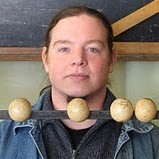## Introduction to Mathematics 1

 Author: Offering Institution: Technische Hochschule Lübeck Course Language: English Field of Knowledge: Preparation Courses Free of Charge Average Workload: 75 Hours Free of Charge

## What awaits you in this course?

In this online course Introduction to mathematics 1, you will obtain basic knowledge of Mathematics, we cover the most important aspects of the world of numbers and logic. With many facts that will open up new insights into exciting sciences.## What can you learn in this course?

After completing the course „Introduction to Mathematics 1“:

• You can read and write the set notation correctly; interpret expressions with sets and set operations correctly;
• Compose sets from predefined basic quantities using set operations;
• Convert quantity diagrams into quantity expressions and vice versa;
• Calculate binomial coefficients correctly by hand.
• you can define functions with domain and value set and check for correctness;
• you can construct simple functions with function rules, domain and value range;
• you know the relationship between function and inverse function and can check these for simple examples;
• you can check in simple cases whether a given function is reversible.
• You can analyze logical formulas for syntactical correctness,
• interpret logical formulas and determine their truth value,
• formulate simple facts in the language of propositional logic.
• you will learn how certain methods of mathematical verifying can be justified by using propositional logic and
• you will learn how digital combinational circuits can be designed using propositional logic.

## Outline

1. Sets
2. Relations and Functions
3. Components of Propositional Logic
4. Laws of Propositional Logic
5. Applications of the Propositional Logic

## Further authorsKai Obermüller
Kai has studied mathematics and chemistry and he currently works also as an author for MOOCs on oncampus.de. He has been employing in Massive Open Online Courses for over five years, started there as a participant. For more than two years he has also been creating courses and content at the oncampus for the learners. His interests are mainly in the field of science and making.